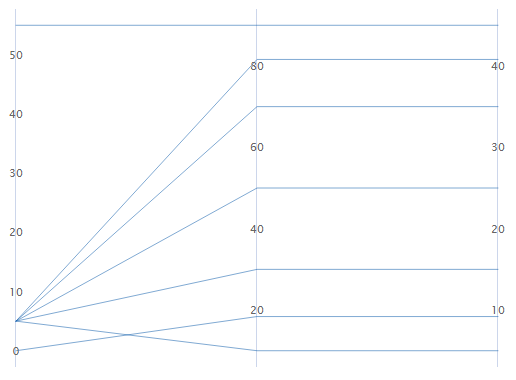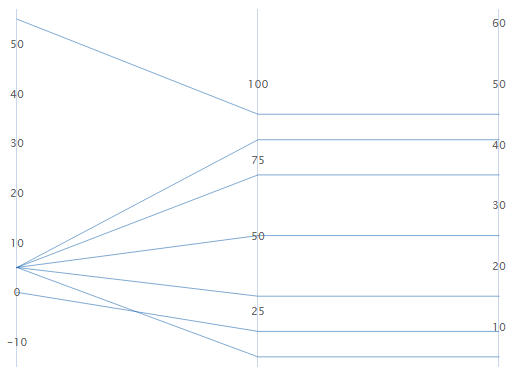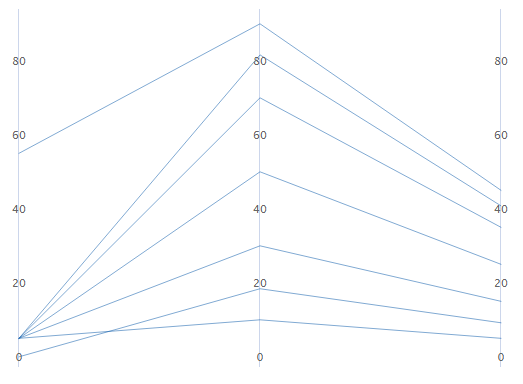# Select the type of y-scale

Graph > Parallel Coordinates Plot

From the Y-scale based on the following drop down, select how you want to display the y-scale.

## Percent of range

Select to plot each variable with a unique y-scale. A series that contains all of the minimum or maximum values for each variable will be a horizontal line.## Standard units

Select to plot each variable with a unique y-scale. The minimum and maximum values for each scale are the overall minimum and maximum z-score values from all the data that you entered converted to each variable's scale.

For example, the first variable's maximum value has a z-score of 2, while the other two variable's maximum value has a z-score of 1. The maximum value for each variable's y-scale is the value that corresponds to a z-score of 2.## Original data

Select to use a single y-scale that is repeated for each variable. The minimum and maximum values for the scale are the overall minimum and maximum values from all the data that you entered.## Sort variables from greatest to least variation in lines

If you select Standard units or Original data, you can sort the variables based on the variation. This can be useful when you have many variables and you want to see which one separates the series the most. If you do not select this option, Minitab sorts the columns in the same order as you enter them in the Variables dialog box.